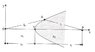# Show the Solution to a Cartesian Oval Homework Problem

• fluidistic
In summary, the problem involves finding the equation of an interface curve using the given information about the points S and P. The attempt at a solution involves using the concept of constant time and the velocity of light in vacuum to determine the equation. The solution was found using this approach.

Gold Member

## Homework Statement

See the picture for the situation of the problem.
I'm told that any ray starting from S and getting through the "Cartesian Oval" reach point P.
I must show that the equation of the interface curve is $$l_0n_1+l_i n_2=K$$ where K is a constant.
So far I've showed that $$l_0=\sqrt {x^2+y^2}$$ and $$l_i=\sqrt {y^2+(s_0 + s_i -x)^2}$$. But I remain stuck as how to proceed further.
Any idea is greatly appreciated.

## Homework Equations

Snell's law? I've tried something with it but didn't reach anything.
Maybe Fermat's principle?

## The Attempt at a Solution

See above.

#### Attachments

•scan1.jpg
24.8 KB · Views: 417

Since the points S and P are fixed, the total time taken by the light to travel from S to P must be constant.
So t1 = l1/v1 and t2 = l2/v2
Now v1 = C/n1 and v2 = C/n2, where C is the velocity of the light in vacuum.
Hence find t = t1 + t2 = ...?

Last edited:

rl.bhat said:
Since the points S and P are fixed, the total time taken by the light to travel from S to P must be constant.
So t1 = l1/v1 and t2 = l2/v2
Now v1 = C/n1 and v2 = C/n2, where C is the velocity of the light.
Hence find t = t1 + t2 = ...?

Thank you so much! Really bright and not complicated. Yet I totally missed it.
Problem solved!